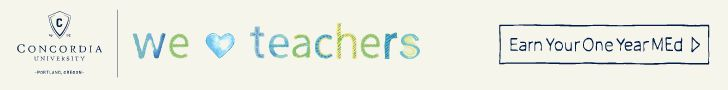# Third-Grade Mathematics StandardsParents of third-graders must support their children as they tackle brand new skills and help to form good study habits. Your child is introduced to math milestones: multiplication, division, and fractions. Here are some examples of the skills he or she will practice in third-grade math, along with useful questions to ask your son or daughter’s teacher, and strategies for helping him or her learn at home.

### Operations and Algebraic Thinking

1. Understand the meaning of multiplication and how it relates to addition.
6 × 3 = 18 is the same as 6 + 6 + 6 = 18
2. By the end of third grade, learn multiplication tables and use them from memory.
3. Understand division as a separation of numbers into equal groups.
9 ÷ 3 = 3 is the same as separating nine objects into three groups of three
4. Understand how multiplication and division relate to each other.
4 × 5 = 20 ÷ 4
5. Use a letter to represent a whole number in multiplication and division problems.
12 ÷ x = 6

### Number and Operations in Base Ten

1. Use knowledge of place value to add and subtract within 1,000 and to round to the nearest 10.

### Number and Operations: Fractions

1. Understand how fractions with a numerator of 1 — ½, ⅓, ¼ — relate to equal parts of a whole number.
2. Compare equal fractions (such as ½ and 2/4) using number lines or visual models.
¼ + ¼ = 2/4 which equals ½ of the circle, so 2/4 = ½### Measurements and Data

1. Solve problems by measuring object mass and liquid volume.
2. Use picture and bar graphs to illustrate measurement data.

### Geometry

1. Find the area of shapes.
Q: Find the area of the rectangle.A: 12 ft x 10 ft = 120 ft2
So the area of the rectangle is 120 ft2.
2. Relate fractions to shapes as they divide a whole into equal parts.
Q: Show the fraction 1/6 on a circle.
A:Learn More: Click to view related resources.